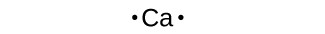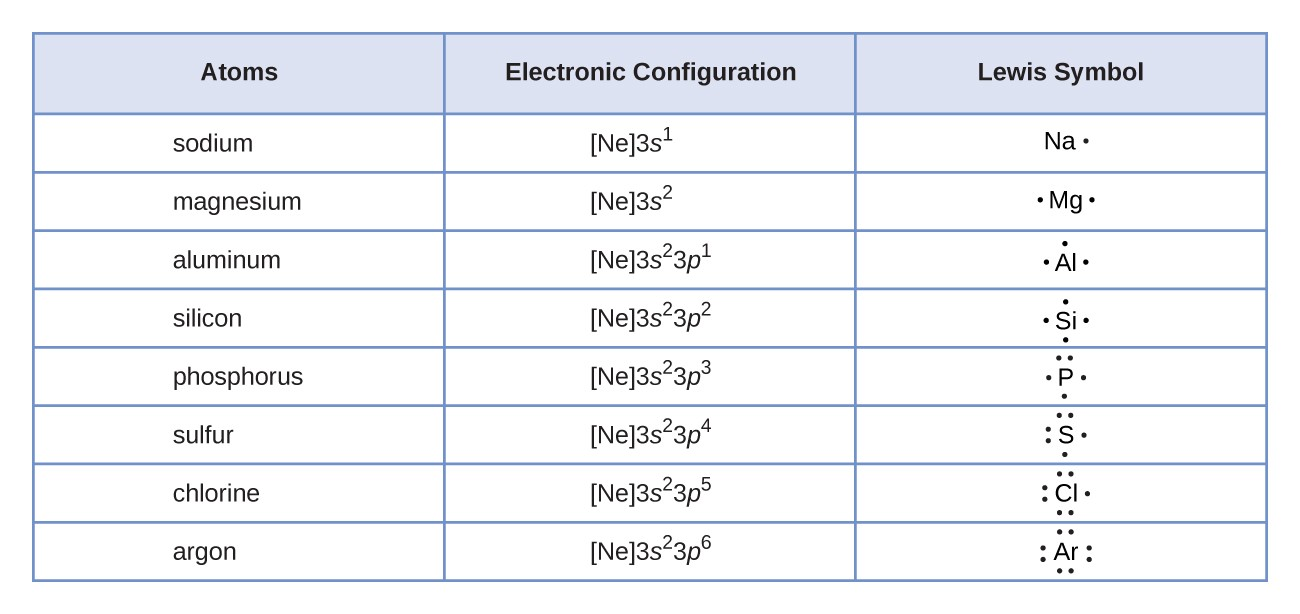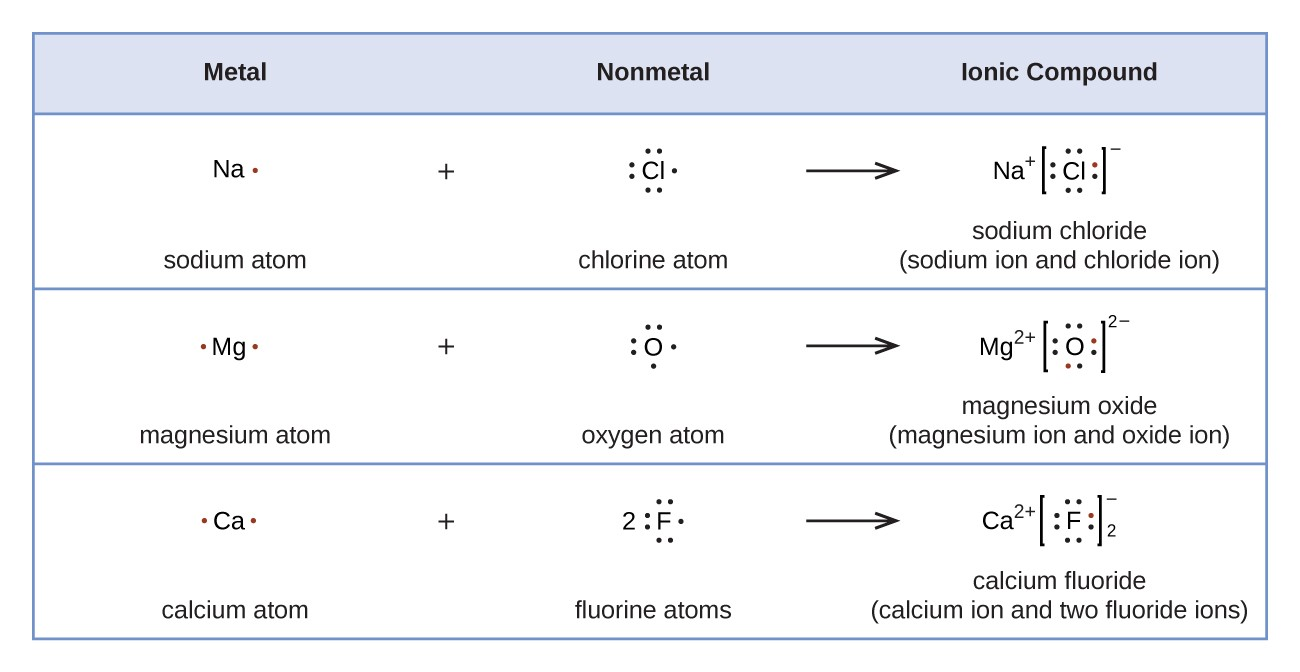## 6.1 Lewis Electron Dot Symbols

### Learning Objectives

By the end of this section, you will be able to:

• Write Lewis symbols for neutral atoms and ions

## Lewis Symbols of Monoatomic Elements

In almost all cases, chemical bonds are formed by interactions of valence electrons in atoms. To facilitate our understanding of how valence electrons interact, a simple way of representing those valence electrons would be useful.

A Lewis electron dot symbol (or electron dot diagram or a Lewis diagram or a Lewis structure) is a representation of the valence electrons of an atom that uses dots around the symbol of the element. The number of dots equals the number of valence electrons in the atom. These dots are arranged to the right and left and above and below the symbol, with no more than two dots on a side. (It does not matter what order the positions are used.)

For example, the Lewis electron dot symbol for calcium is simplyFigure 1 shows the Lewis symbols for the elements of the third period of the periodic table.Figure 1. Lewis symbols illustrating the number of valence electrons for each element in the third period of the periodic table.

Lewis symbols can also be used to illustrate the formation of cations from atoms, as shown here for sodium and calcium:Likewise, they can be used to show the formation of anions from atoms, as shown below for chlorine and sulfur:Figure 2 demonstrates the use of Lewis symbols to show the transfer of electrons during the formation of ionic compounds.Figure 2. Cations are formed when atoms lose electrons, represented by fewer Lewis dots, whereas anions are formed by atoms gaining electrons. The total number of electrons does not change.

### Example 1: Writing Lewis DoT SYmbols of Elements

What is the Lewis electron dot symbol for each element?

1. aluminum
2. selenium

What is the Lewis electron dot symbol for each element?

1. phosphorus
2. argon

### Example 2: Writing Lewis DoT SYmbols of Ions

What is the Lewis electron dot symbol for each ion?

1. Ca2+
2. O2−

The valence electron configuration of thallium, whose symbol is Tl, is 6s25d106p1. What is the Lewis electron dot diagram for the Tl+ ion?

### Key Takeaways

• Lewis electron dot diagrams use dots to represent valence electrons around an atomic symbol.
• Lewis electron dot diagrams for ions have fewer (for cations) or more (for anions) dots than the corresponding atom.

### Exercises

1.  Explain why the first two dots in a Lewis electron dot symbol are drawn on the same side of the atomic symbol.

2.  Is it necessary for the first dot around an atomic symbol to go on a particular side of the atomic symbol?

3.  What column of the periodic table has Lewis electron dot symbol with two electrons?

4.  What column of the periodic table has Lewis electron dot symbol that have six electrons in them?

5.  Draw the Lewis electron dot symbol for each element.

a)  strontium

b)  silicon

6.  Draw the Lewis electron dot symbol for each element.

a)  krypton

b)  sulfur

7.  Draw the Lewis electron dot symbol for each element.

a)  titanium

b)  phosphorus

8.  Draw the Lewis electron dot symbol for each element.

a)  bromine

b)  gallium

9.  Draw the Lewis electron dot symbol for each ion.

a)  Mg2+

b)  S2−

10.  Draw the Lewis electron dot symbol for each ion.

a)  In+

b)  Br

11.  Draw the Lewis electron dot symbol for each ion.

a)  Fe2+

b)  N3−

12.  Draw the Lewis electron dot symbol for each ion.

a)  H+

b)  H